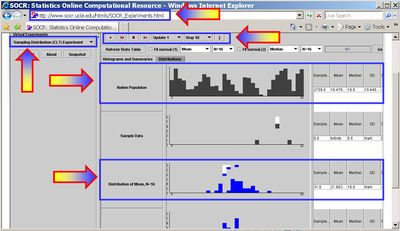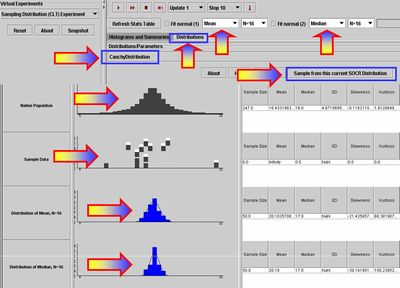# SOCR EduMaterials Activities GeneralCentralLimitTheorem2

## Summary

This activity represents a second general demonstration of the effects of the Central Limit Theorem (CLT). The first such activity is SOCR EduMaterials Activities GeneralCentralLimitTheorem. The activity is based on the SOCR Sampling Distribution CLT Experiment. This experiment builds upon a RVLS CLT applet by extending the applet functionality and providing the capability of sampling from any SOCR Distribution

## The SOCR CLT Experiment

To start this Experiment, go to SOCR Experiments and select the SOCR Sampling Distribution CLT Experiment from the drop-down list of experiments in the left panel. The image below shows the interface to this experiment. The main control widgets on this image are boxed in blue and pointed to by arrows. The generic control buttons on the top allow you to do one or multiple steps/runs, stop and reset this experiment. The two tabs in the main frame provide graphical access to the results of the experiment (Histograms and Summaries) or the Distribution selection panel (Distributions). Remember that choosing sample-sizes <= 16 will animate the samples (second graphing row), whereas larger sample-sizes (N>20) will not show the updated sampling distributions (bottom two graphing rows).## Exercise 1

Above we show a hand-generated distribution that one can construct by clicking and dragging the mouse in the row 1 graphing panel (native process distribution). Hint: Bring the mouse over various components of the applet to see tool-tip description of each of these widgets.

• Can you think of a process that may have this distribution?
• Now draw a random sample of size 20 from this distribution.
• What is the mean of this sample (look in row 2)? What is the corresponding standard deviation?
• What does the distribution of this sample look like? What would change if we increased the sample size to 100 or 1000? Try it and think of a reason for that phenomenon. (This point helps demonstrate that the distribution of the sample will tend to look like the parent distribution, as the sample-size increases).
• Now let's go back and draw many samples of size 20, again from the same distribution and compute the mean of each of them. If we draw 100 samples each of size 20 how many sample means do I have? Their distribution is called the sampling distribution of the sample mean. Similary, we can construct the sampling distributions for other parameters (e.g., median, variance, range, etc.
• What does the distribution of the sample mean (row 3) look like? Does it look like the one in the second row? Does it depend on the sample-size?
• Now, compare the distribution of the last sample and the sampling distribution of the sample mean. What do you conclude? Why?
• Compare also the mean and standard deviations of the graphing panels in rows 2 and 3. Repeat the exercise with another distribution (selected from the drop-down list of distributions in the second tab-panel in the main window, or drawn by hand directly in the top row graphing canvas).
• Can we make the same conclusion as before? Do you think that if we try another distribution you will come to the same conclusion? Try it and answer empirically. Pick up your favorite distribution, and then try with your least favorite one.
• Below, we have shown the outcome of running the experiment a number of times with the population mean and median as parameters of interest. Notice the sampling distributions of the sample average and the sample median.